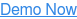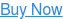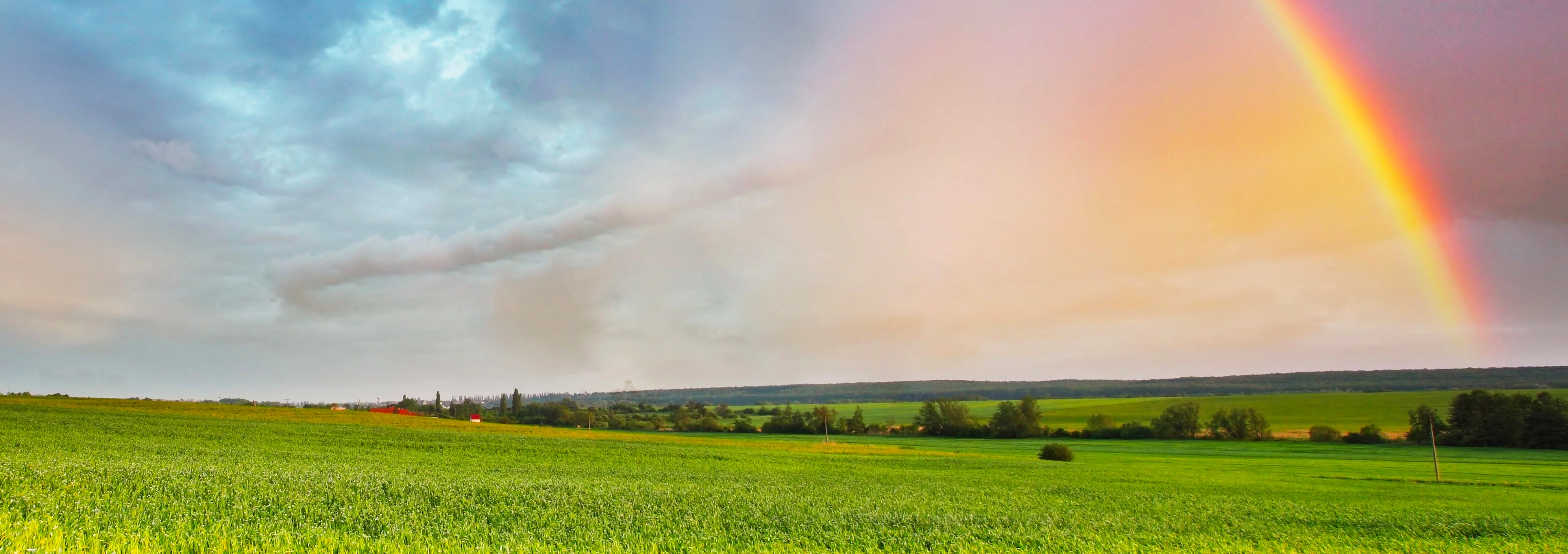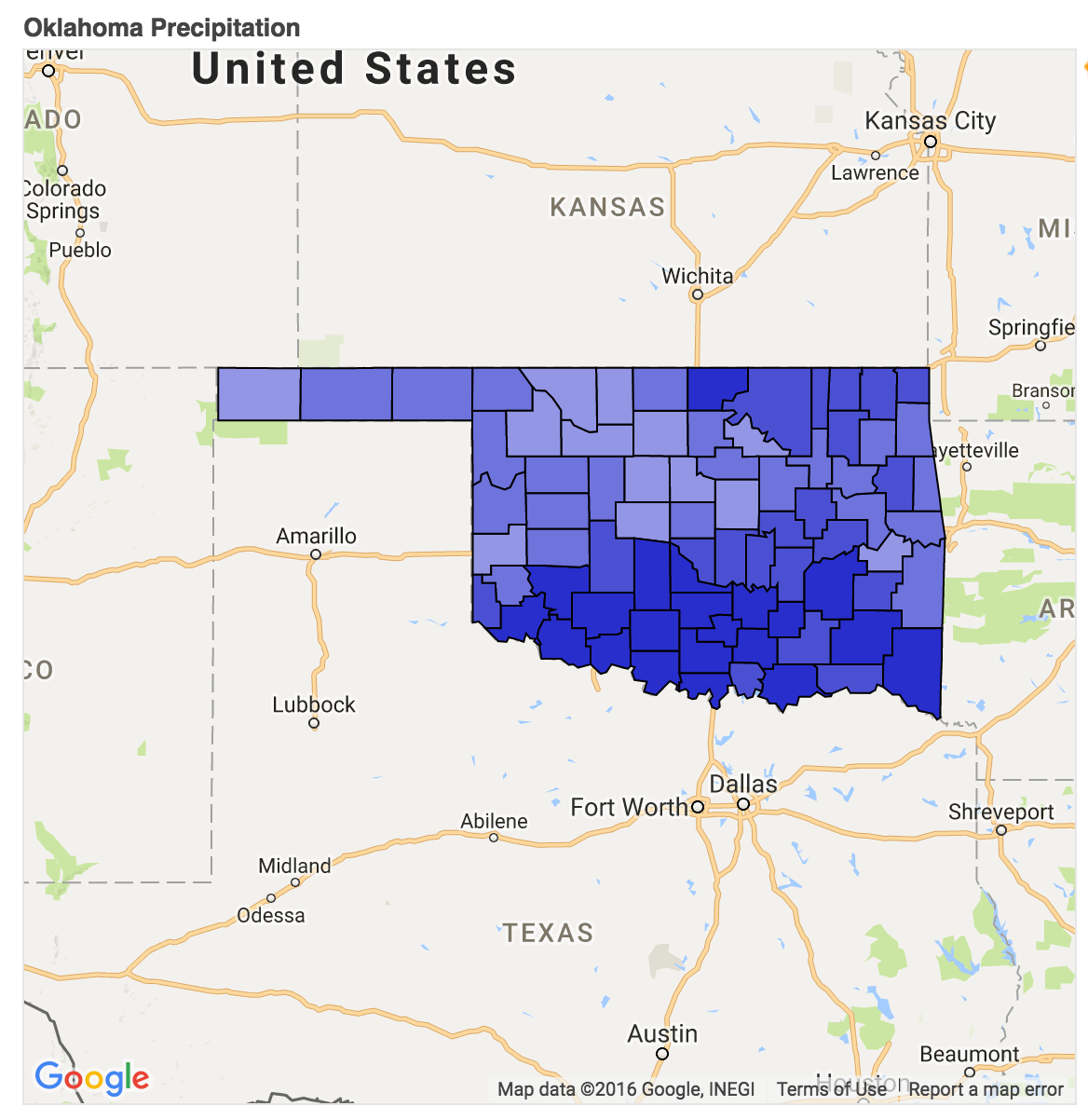# Assets: Creation and Display

by Justin Stewart, on Sep 7, 2016 1:28:12 PM

In this tutorial, we're going to create assets from geojson for every county in Oklahoma using Insight API's Python client library. Then, we're going to walk through creating a choropleth map using Bokeh.

We'll accomplish this by writing several small, lightweight scripts.

## Getting Started

Let's start off by creating a clean working directory and virtualenv:

 ```mkdir tutorial && cd tutorial virtualenv venv && source venv/bin/activate pip install skywise-insight arrow bokeh geojson```
`Then, we'll download and unpack our county geojson files: `
 ```curl -L https://github.com/wdtinc/Insight-API/raw/master/static/data/ok_counties.zip > ok_counties.zip unzip ok_counties.zip -d data```
` NOTE: If you haven't already, set up your environment to work with the Insight Python client.`

Let's write a script called `create_assets.py` that will use our county geojson files to create Assets:

 ```import geojson import json from glob import glob from skywiseinsight import Asset def create_asset(gj_filename): # Read County GeoJSON with open(gj_filename, 'r') as f: gj = geojson.loads(f.read()) # Create and Save Asset asset = Asset() asset.description = gj['features']['properties']['name'] asset.shape = gj['features']['geometry'] asset.save() return asset def main(): asset_ids = [] # Create Assets for filename in glob('./data/*geo.json'): asset = create_asset(filename) asset_ids.append(asset.id) print "%s: %s" % (asset.id, asset.description) # Write Asset IDs to JSON File with open('asset_ids.json', 'w') as f: asset_ids_json = json.dumps({ 'asset_ids': asset_ids }) f.write(asset_ids_json) if __name__ == '__main__': main()```
` `

NOTE: `asset.shape` will accept any valid geojson Polygon/Multipolygon dictionary. You can also use the Polygon and Multipolygon geometry classes provided by the geojson module if it is more convenient for your use case.

We should now have an `asset_ids.json` file containing IDs for our newly created assets. These will be required for the next part of the tutorial.

## Retrieving County Precip

Now, we'll create a script called `load_asset_data.py` that will gather precipitation data for Q2 2016 for each of our counties:

 ```import arrow import json from skywiseinsight import Asset, DailyPrecipitation start = arrow.get('2016-04-01').datetime end = arrow.get('2016-06-30').datetime def main(): with open('asset_ids.json' ,'r') as f: assets_ids_json = json.loads(f.read()) # Gather Assets assets = [] for asset_id in assets_ids_json['asset_ids']: asset = Asset.find(asset_id) assets.append(asset) # Collect Precipitation Data asset_precip = [] for asset in assets: precip = DailyPrecipitation.asset(asset.id, start=start ,end=end) average_precip = precip.accumulationStatistics['mean'] asset_precip.append({ 'id': asset.id, 'average_precip': average_precip, 'shape': asset.shape }) print "%s: %.2f mm" % (asset.description, average_precip) # Write Precip Data to JSON File with open('asset_data.json', 'w') as f: asset_data_json = json.dumps({ 'assets': asset_precip }) f.write(asset_data_json) if __name__ == '__main__': main()```
` `

NOTE: We're only using mean precipitation in this example, but `DailyPrecipitation.asset(..)` retrieves min, max, and time series data over the entire quarter for our asset. To check it out in this example, add `print precip.json()` immediately after.

Our resulting file contains average precipitation and the shapes of our counties: everything we need to create our map.

## Create a Bokeh Map

Our last script will be called `bokeh_display.py`

### The Base Map

We'll start off by using Bokeh's GMapPlot to create a base map of Oklahoma.

NOTE: This step of the tutorial requires a Google Maps API Key. Get one here if you haven't already.

` `
 ```# Imports from bokeh.io import output_file, show from bokeh.models import GMapPlot, GMapOptions, DataRange1d def create_plot(): map_options = GMapOptions(lat=35.0078, lng=-99.0929, map_type="satellite", zoom=6) plot = GMapPlot(x_range=DataRange1d(), y_range=DataRange1d(), map_options=map_options, api_key="{YOUR_MAPS_API_KEY}") plot.title.text = "Oklahoma Precipitation" return plot def main(): # Create Plot plot = create_plot() # Display Plot output_file("county_map.html") show(plot) if __name__ == '__main__': main()```
` `

You should be able to open up the newly created `county_map.html` to see our map of Oklahoma.

### Plot and Color Your Assets

Time to add counties to the map using Bokeh Patch Glyphs. This step will create a patch and associate a color value with it based on the value of the asset's average precipitation:

` `
 ```# Imports ... import json from bokeh.models import ColumnDataSource, Patch from collections import OrderedDict def create_plot(): ... # Color Map: Key values are in millimeters color_coding = OrderedDict(sorted({ 100: '#DBDCF6', 150: '#B7B9ED', 200: '#9396E5', 250: '#6F73DC', 300: '#4B50D3', 350: '#282ECB' }.items(), key=lambda t: t)) def plot_asset(plot, asset): def color(value): """ Returns the color range for your value.""" color = '#FFFFFF' for k, v in color_coding.items(): if value > k: color = v return color # The Datasource Tells Bokeh How to Plot Our Assets lat = [c for c in asset['shape']['coordinates']] lon = [c for c in asset['shape']['coordinates']] source = ColumnDataSource(data=dict( lat=lat, lon=lon )) patch = Patch(x="lon", y="lat", fill_color=color(asset['average_precip'])) plot.add_glyph(source, patch) def main(): # Create Plot ... # Load Asset Glyphs with open('asset_data.json', 'r') as f: asset_json = json.loads(f.read()) for asset in asset_json['assets']: plot_asset(plot, asset) # Display Plot ... if __name__ == '__main__': main()```
` `

Your final result should look like this:## Next Steps

You can add other effects to your map with Bokeh if you want to take this tutorial further. In particular, you might look at creating a Hover Effect to display county precip values.

Topics:SkyWiseDevelopers